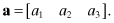APPENDIX ASUMMARY OF VECTOR/MATRIX OPERATIONS

This Appendix summarizes properties of vector and matrices, and vector/matrix operations that are often used in estimation. Further information may be found in most books on estimation or linear algebra; for example, Golub and Van Loan (1996), DeRusso et al. (1965), and Stewart (1988).

A.1 DEFINITION

A.1.1 Vectors

A vector is a linear collection of elements. We use a lower case bold letter to denote vectors, which by default are assumed to be column vectors. For example,is a three-element column vector. A row vector is (obviously) defined with elements in a row; for example,A vector is called unit or normalized when the sum of elements squared is equal to 1:for an n-element vector a.

A.1.2 Matrices

A matrix is a two-dimensional collection of elements. We use bold upper case letters to denote matrices. For example,is a matrix with two rows and three columns, or a 2 × 3 matrix. Individual elements are labeled with the first subscript indicating the row, and the second indicating the column. An n-element column vector may be ...

Get Advanced Kalman Filtering, Least-Squares and Modeling: A Practical Handbook now with the O’Reilly learning platform.

O’Reilly members experience books, live events, courses curated by job role, and more from O’Reilly and nearly 200 top publishers.